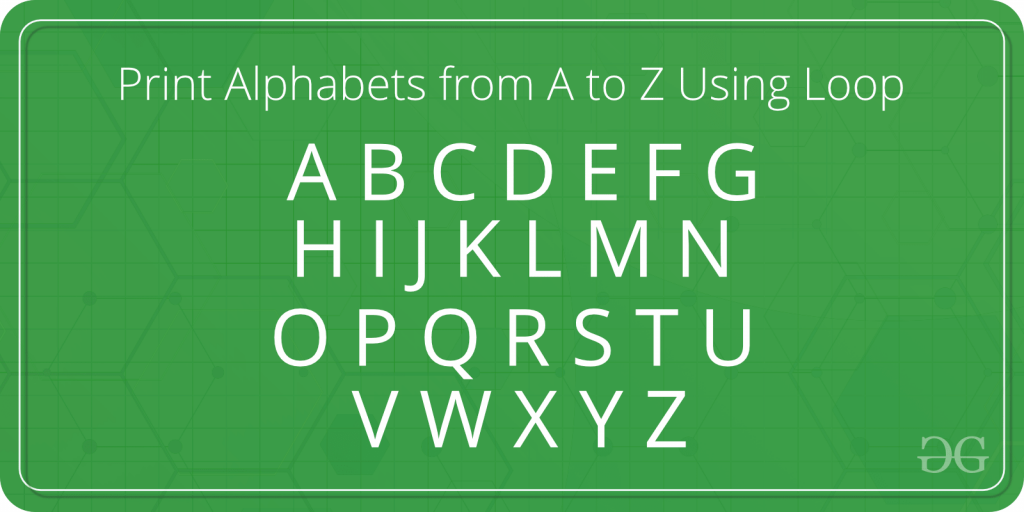# JavaScript program to print Alphabets from A to Z using Loop

Our task is to print the alphabet from A to Z using loops. In this article, we will mainly focus on the following programs and their logic:

• Using for loop: The elements are iterated through a predetermined number of times in the JavaScript for a loop.
• Using the while loop: the element is iterated an infinite number of times. if a number of iterations are not given.
• Using a do-while loop : Similar to a while loop iterates the element an infinite of times .however whether the condition is true or false, the code is always run at least once.#### Program to print (A to Z) and (a to z) using for loop:

In the below program:

• For loop is used to print the alphabet from A to Z. A loop variable is taken to do this of type ‘char’.
• The loop variable ‘i’ is initialized with the first alphabet ‘A’ and incremented by 1 on every iteration.
• In the loop, the character ‘i’ is printed as the alphabet.

Example: Below is the example that will print (A to Z) and (a to z) using for loop:

## Javascript

 `// Declaring Variables` `let i;`   `console.log(``"Alphabets form (A-Z) are:"``);`   `// Using for loop for (A-Z):` `for` `(i = 65; i <= 90; i++) {` `    ``console.log(String.fromCharCode(i));` `}`   `console.log(``"Alphabets from (a-z) are:"``);`   `// Using for loop for (a-z):` `for` `(i = 97; i <= 122; i++) {` `    ``console.log(String.fromCharCode(i));` `}`

Output:

```Alphabets form (A-Z) are:
A B C D E F G H I J K L M N O P Q R S T U V W X Y Z
Alphabets from (a-z) are:
a b c d e f g h i j k l m n o p q r s t u v w x y z```

#### Program to print (A to Z) and (a to z) using while loop:

In the below program:

• While loop is used to print the alphabet from A to Z. A loop variable is taken to display of type ‘char’.
• The loop variable ‘i’ is initialized with the first alphabet ‘A’ and incremented by 1 on every iteration.
• In the loop, the character ‘i’ is printed as the alphabet.

Example: Below is the example that will print (A to Z) and (a to z) using for while loop:

## Javascript

 `// Declaring Variables` `let i;`   `// Initializing i = 65 for Uppercase:` `i = 65;` `console.log(``"Alphabets form (A-Z) are:"``);`   `while` `(i <= 90) {` `    ``console.log(String.fromCharCode(i));` `    ``i++;` `}`   `i = 97;` `console.log(``"Alphabets from (a-z) are:"``);`   `while` `(i <= 122) {` `    ``console.log(String.fromCharCode(i));` `    ``i++;` `}`

Output:

```Alphabets form (A-Z) are:
A B C D E F G H I J K L M N O P Q R S T U V W X Y Z
Alphabets from (a-z) are:
a b c d e f g h i j k l m n o p q r s t u v w x y z```

#### Program to print (A to Z) and (a to z) using a do-while loop:

In the below program:

• The do-while loop is used to print the alphabet from A to Z. A loop variable is taken to display of type ‘char’.
• The loop variable ‘i’ is initialized with the first alphabet ‘A’ and incremented by 1 on every iteration.
• In the loop, the character ‘i’ is printed as the alphabet.

Example: Below is the example that will print (A to Z) and (a to z) using for do-while loop:

## Javascript

 `// Declaring Variables` `let i;`   `// Initializing i = 65 for Uppercase:` `i = 65;` `console.log(``"Alphabets form (A-Z) are:"``);`   `do` `{` `    ``console.log(String.fromCharCode(i));` `    ``i++;` `} ``while` `(i <= 90);`   `i = 97;` `console.log(``"Alphabets from (a-z) are:"``);`   `do` `{` `    ``console.log(String.fromCharCode(i));` `    ``i++;` `} ``while` `(i <= 122);`

Output:

```Alphabets form (A-Z) are:
A B C D E F G H I J K L M N O P Q R S T U V W X Y Z
Alphabets from (a-z) are:
a b c d e f g h i j k l m n o p q r s t u v w x y z```

Whether you're preparing for your first job interview or aiming to upskill in this ever-evolving tech landscape, GeeksforGeeks Courses are your key to success. We provide top-quality content at affordable prices, all geared towards accelerating your growth in a time-bound manner. Join the millions we've already empowered, and we're here to do the same for you. Don't miss out - check it out now!

Previous
Next